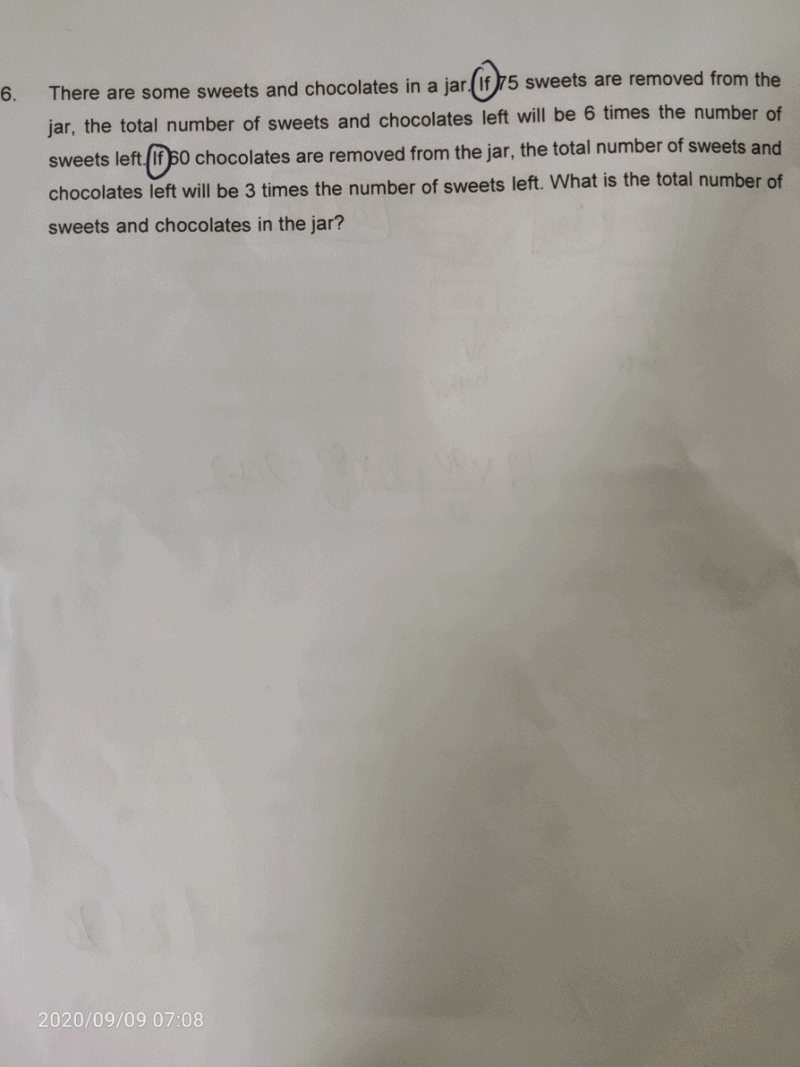# QuestionNumber of sweets → u + 75

Scenario 1

Number of sweets after removing 75 → u

Total number of sweets & chocolates → 6u

⇒ Number of chocolates → 6u – u = 5u

Scenario 2

Number of chocolates after removing 60 → 5u – 60

Total number of sweets & chocolates → (5u – 60) + (u + 75) = 6u + 15

Total number of sweets & chocolates is also 3 times number of sweets

6u + 15 = 3(u + 75)

6u + 15 = 3u + 225

3u = 210

1u = 70

Actual total number of sweets & chocolates → 5u + (u + 75) = (6 × 70) + 75 = 495

0 Replies 0 Likes